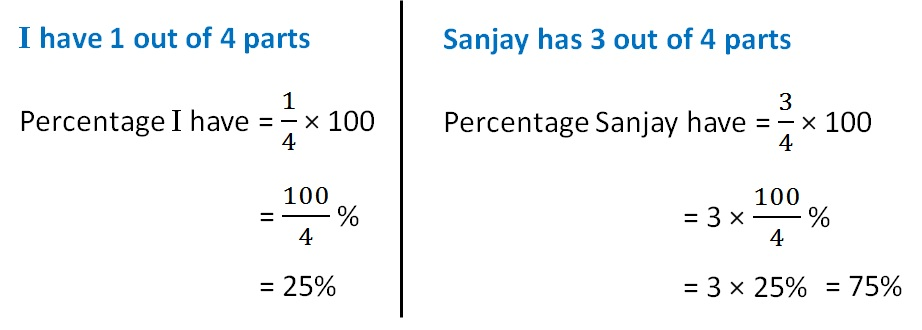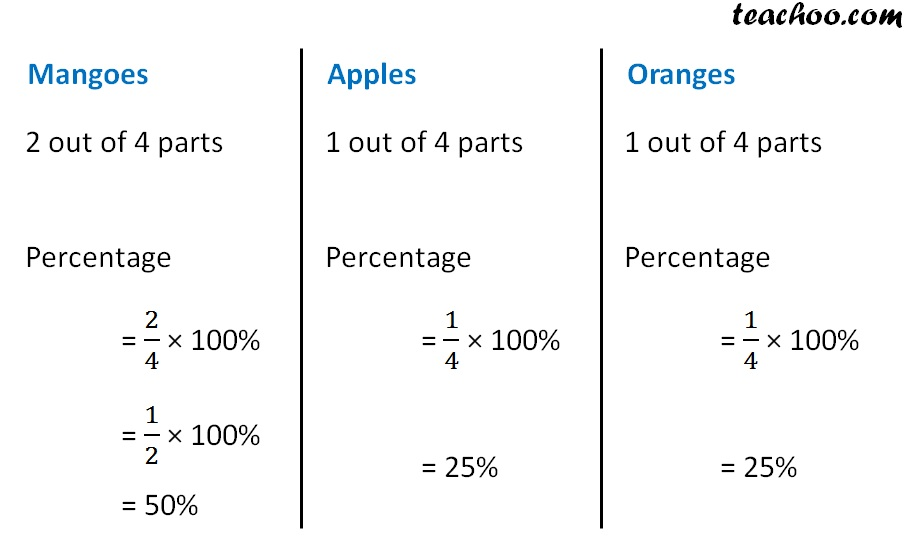Ratios to percentage

Chapter 8 Class 7 Comparing Quantities
Concept wise

#### Suppose the ratio of money I & Sanjay have is 1 : 3. What percentage to total money do I have, & what percentage does Sanjay have?

Given ratio is 1 : 3

So, 1 part I have, 3 Parts Sanjay has

Total parts = 1 + 3  = 4

So,#### Suppose the ratio of Mangoes, Apples and Oranges I have is 2 : 1 : 1. How much percent mangoes, apples, Oranges do I have?

Given ratio is 2 : 1 : 1

So, There are 2 parts of mangoes, 1 part of Apples & 1 part of oranges.

Total parts = 2 + 1 + 1 = 4Get live Maths 1-on-1 Classs - Class 6 to 12

### Transcript

I have 1 out of 4 parts Percentage I have = 1/4 × 100 = 100/4 % = 25% Sanjay has 3 out of 4 parts Percentage Sanjay have = 3/4 × 100 = 3 × 100/4 % = 3 × 25% Mangoes 2 out of 4 parts Percentage = 2/4 × 100% = 1/2 × 100% = 50% Apples 1 out of 4 parts Percentage = 1/4 × 100% = 1/2 × 100% = 25% Oranges 1 out of 4 parts Percentage = 1/4 × 100% = 1/2 × 100% = 25%